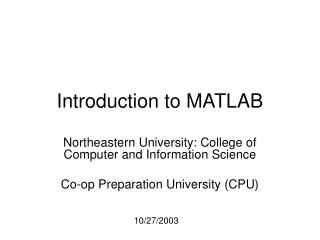DownloadDownload PresentationIntroduction to MATLAB

Introduction to MATLAB

Télécharger la présentationIntroduction to MATLAB

- - - - - - - - - - - - - - - - - - - - - - - - - - - E N D - - - - - - - - - - - - - - - - - - - - - - - - - - -
Presentation Transcript

1. Introduction to MATLAB Northeastern University: College of Computer and Information Science Co-op Preparation University (CPU) 10/27/2003

2. Overview for 10/29/2003 • Brief review of topics covered in last session (10/29/2003) • File Output • MATLAB Scripts and Functions

3. Review for 10/27/2003 • Plotting – in depth • File input • Wing’s birthday, or something.

4. Plotting • Several types of plots available • Plot • Polar • Bar • Hist

5. Color options • Color options: • Yellow - ‘y’ • Magenta - ‘m’ • Cyan - ‘c’ • Red - ‘r’ • Green - ‘g’ • Blue - ‘b’ • White - ‘w’ • Black - ‘k’ • Example: • plot(temp, ‘y’);

6. Line options • Line styles: • - solid line (default) • -- dashed line • : dotted line • -. dash-dot line

7. Marker Options • + - plus sign • o - circle • * - asterisk • . - Point • x - cross • s - square • d - diamond • ^ - upward pointing triangle • v - downward pointing triangle • > - right pointing triangle • < - left pointing triangle • p - five-pointed star (pentagram) • h - six-pointed star (hexagram)

8. Plot() (from MATLAB help) • Linear 2-D plot • Syntax: • plot(Y) • plot(X1,Y1,...) • plot(X1,Y1,LineSpec,...) • plot(...,'PropertyName',PropertyValue,...) • h = plot(...)

9. Plot() con’t: 2 • MATLAB defaults to plotting a blue line between points • Other options exist: • Different color lines • Different types of lines • No line at all!

10. Polar() • Plot polar coordinates • Syntax: • polar(theta,rho) • polar(theta,rho,LineSpec) • Theta – Angle counterclockwise from the 3 o’clock position • Rho – Distance from the origin

11. Polar() con’t: 2 • Line color, style and markings apply as they did in the example with Plot().

12. Bar() • Creates a bar graph • Syntax • bar(Y) • bar(x,Y) • bar(...,width) • bar(...,'style') • bar(...,LineSpec)

13. Hist() • Creates a histogram plot • Syntax: • n = hist(Y) • n = hist(Y,x) • n = hist(Y,nbins)

14. High-Level File I/O • I/O = input/output • 3 important commands for input • csvread • dlmread • textread

17. textread • Reads formatted data from a text file • Syntax: • [A,B,C,...] = textread('filename','format') • [A,B,C,...] = textread('filename','format',N) • [...] = textread(...,'param','value',...) • Ex. A = textread(‘blah.txt’, ‘%u%u’); • Useful, but try to do without it, MATLAB is somewhat slower when dealing with text data

18. Delimiters • Delimiter: A character or sequence of characters marking the beginning or end of a unit of data. • Ex. 1,2,3 (the delimiter would be ,) • Also 1:2:3 (the delimiter would be :)

19. Delimiters, con’t: 2 • The most common delimiter is a comma: hence the term csv or comma separated values. • Microsoft Excel can read csv formatted files

20. High Level File Output • Some of the input commands have corresponding high-level output commands • csvwrite • dlmwrite

21. csvwrite • Write a matrix to a comma-seperated value file • Syntax: • csvwrite('filename',M) • csvwrite('filename',M,row,col) • Ex. csvwrite(‘blah.csv’,a);

22. dlmwrite • Writes a matrix to a delimited file (using the delimiter you specify) • Syntax: • dlmwrite(filename,M,delimiter) • dlmwrite(filename,M,delimiter,row,col) • Ex. dlmwrite(‘blah.txt’,a,’:’);

23. Low-Level file I/O • fopen • fclose • fprintf • fgetl / fgets

24. fopen • Opens a file and returns the handle to the file object • File_ID = fopen(‘blah.txt’) • Capturing the file handle is necessary to write or read to/from the file

25. fclose • Closes a file associated with a specific file identification handle • Ex. fclose(File_ID); • Ex. fclose(‘all’);

26. fprintf • Multi-use: can output to a file or a screen • Ex. fprintf(fid,'%6.2f %12.8f\n',y); • %6.2f means a floating point with 6 leading decimals and 2 trailing • Specifying 1 instead of fid will output to the screen

27. fgetl / fgets • Get line and get string, respectively. Fgetl will get you a line without the newline character at the end, while fgets will preserve the newline character (\n). • Syntax: • Line = fgetl(File_ID); • Line = fgets(File_ID);

28. Programming in MATLAB • Two types of files: • Scripts • Functions

29. MATLAB Scripts • Scripts are MATLAB commands stored in text files. When you type the name of the script file at the MATLAB prompt the commands in the script file are executed as if you had typed them in from the keyboard. Scripts end with the extension .m • Referred to as M-Files

30. MATLAB functions • Have input and output parameters • MATLAB can return more than one variable at the end of a function • Variables in scope in the MATLAB function go out of scope and are eliminated when the MATLAB function ceases to exist.

31. MATLAB Functions • More on function files tomorrow

32. End